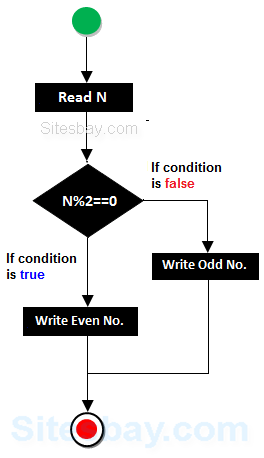# Even or Odd Program in C++

## Even and Odd Program in C++

In general Even numbers are those which are divisible by 2, and which numbers are not divisible 2 is called Odd number.

But in term of programming for find even number we check remainder of number is zero or not, If remainder is equal to zero that means number is divisible by 2. To find remainder of any number we use modulo (%) operator in C language which return remainder as result.### Algorithm for Even and Odd Program in C++

• Step 1: Start
• Step 2: [ Take Input ] Read: Number
• Step 3: Check: If Number%2 == 0 Then
• Print : N is an Even Number.
• Else
• Print : N is an Odd Number.
• Step 4: Exit

## Check give number is Even or Odd

```#include<iostream.h>
#include<conio.h>

void main()
{
int no;
clrscr();
cout<<"Enter any num: ";
cin>>no;
if(no%2==0)
{
cout<<"Even num";
}
else
{
cout<<"Odd num";
}
getch();
}
```

## Output

```Enter any num : 5
Odd num
```

## Example

```#include<iostream.h>
#include<conio.h>

void main()
{
int no;
clrscr();
cout<<"Enter any num : ";
cin>>no;
(no%2==0) ? cout<<"Even num"; : cout<<"Odd num";
getch();
}
```

## Output

```Enter any num : 6
Even num
```

## Example

```#include<iostream.h>
#include<conio.h>

int main()
{
int num;
clrscr();
cout<<"Enter any num: ";
cin>>num;
if(num & 1)
{
cout<<num<<" is odd";
}
else
{
cout<<num<< <<" is even";
}
getch();
}
```

## Output

```Enter any num: 20
20 is even
```

## Pure VPN Privide Lowest Price VPN Just @ \$1.65. Per Month with Non Detected IP Lowest Price Non Detected IP VPN